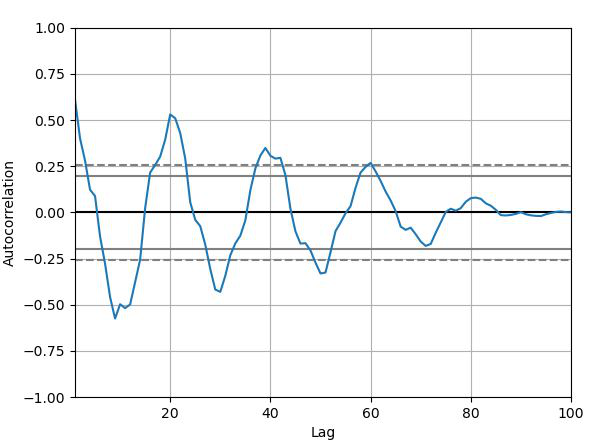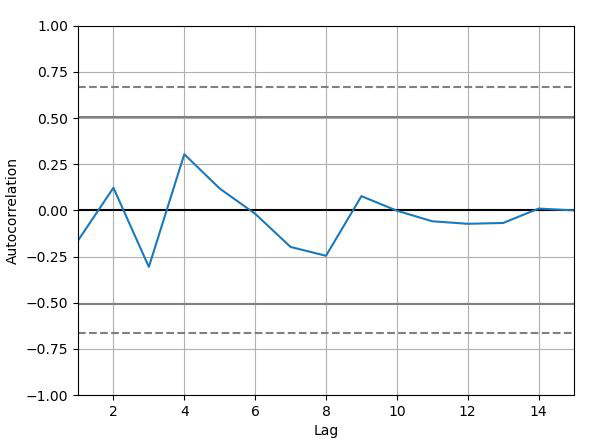# Python Pandas – Plotting the Autocorrelation Plot

• Last Updated : 14 Jan, 2022

Pandas can be used to plot the Autocorrelation Plot on a graph. Plotting the Autocorrelation Plot on a graph can be done using the autocorrelation_plot() method of the plotting module. This function generates the Autocorrelation plot for time series.

## Autocorrelation plot

Autocorrelation plots are a commonly used tool for checking randomness in a data set. This randomness is ascertained by computing autocorrelation for data values at varying time lags. It shows the properties of a type of data known as a time series. These plots are available in most general-purpose statistical software programs. It can be plotted using the pandas.plotting.autocorrelation_plot().

Syntax: pandas.plotting.autocorrelation_plot(series, ax=None, **kwargs)

Parameters:

• series: This parameter is the Time series to be used to plot.
• ax: This parameter is a matplotlib axes object. Its default value is None.

Returns: This function returns an object of class matplotlip.axis.Axes

Example 1:

## Python3

 `# importing various package` `import` `pandas as pd` `import` `numpy as np` `import` `matplotlib.pyplot as plt`   `# making Time series` `spacing ``=` `np.linspace(``-``5` `*` `np.pi, ``5` `*` `np.pi, num``=``100``)` `s ``=` `pd.Series(``0.7` `*` `np.random.rand(``100``) ``+` `0.3` `*` `np.sin(spacing))`   `# Creating Autocorrelation plot` `x ``=` `pd.plotting.autocorrelation_plot(s)`   `# plotting the Curve` `x.plot()`   `# Display` `plt.show()`

Output:Example 2:

## Python3

 `# importing various package` `import` `pandas as pd` `import` `numpy as np` `import` `matplotlib.pyplot as plt`   `# making Time series` `data ``=` `np.array([``12.0``, ``24.0``, ``7.``, ``20.0``, ` `                 ``7.0``, ``22.0``, ``18.0``,``22.0``, ` `                 ``6.0``, ``7.0``, ``20.0``, ``13.0``, ` `                 ``8.0``, ``5.0``, ``8``])`   `# Creating Autocorrelation plot` `x ``=` `pd.plotting.autocorrelation_plot(data)`   `# plotting the Curve` `x.plot()`   `# Display` `plt.show()`

Output:My Personal Notes arrow_drop_up
Recommended Articles
Page :